# Weighted Least Squares Regression

In this example, a weighted least squares regression is applied to a data set containing weighted census data to show the relationship between both the age and education level of a worker and that person's income.

The weighted least squares regression, using the 1010data function `g_wlsq(G;S;Y;W;XX)`, is applied to the Census Income Data Set, which contains weighted census data extracted from the 1994 and 1995 Current Population Surveys conducted by the U.S. Census Bureau.

The analysis uses the following 2 variables in that data set as predictors:
• `age`
• `edu_year`

It also uses the square of the age, which we calculate in this tutorial.

For the weight, we will use the column `instance_weight`, which represents how each person in the survey relates demographically to the overall population.

As a response, the column `wage_per_hour` is used.

After applying the weighted least squares technique, the results show the linear relationship between both the age and education level of the worker and that person's wages per hour.

This analysis will follow the following steps:
• Select only those rows where wages are not equal to zero, since we only want to do the regression for those people who have a job.
• Check the relationship of each of the predictors to the response and adjust for those that have a quadratic form.
• Fit the model on the three predictors and the response.
• Obtain the predicted value of the linear model.
• Obtain the coefficients of the linear model.
• Obtain the p-values of the coefficients.
• Obtain various statistics for the model such as the degrees of freedom, residual sum of squares, mean squared error, and number of observations.
• Calculate the AIC.
• Visualize the results of the weighted least squares regression by plotting the age against both the wages per hour and the predicted value of the linear model.
1. Open the Census Income data set (pub.demo.mleg.uci.censusincome).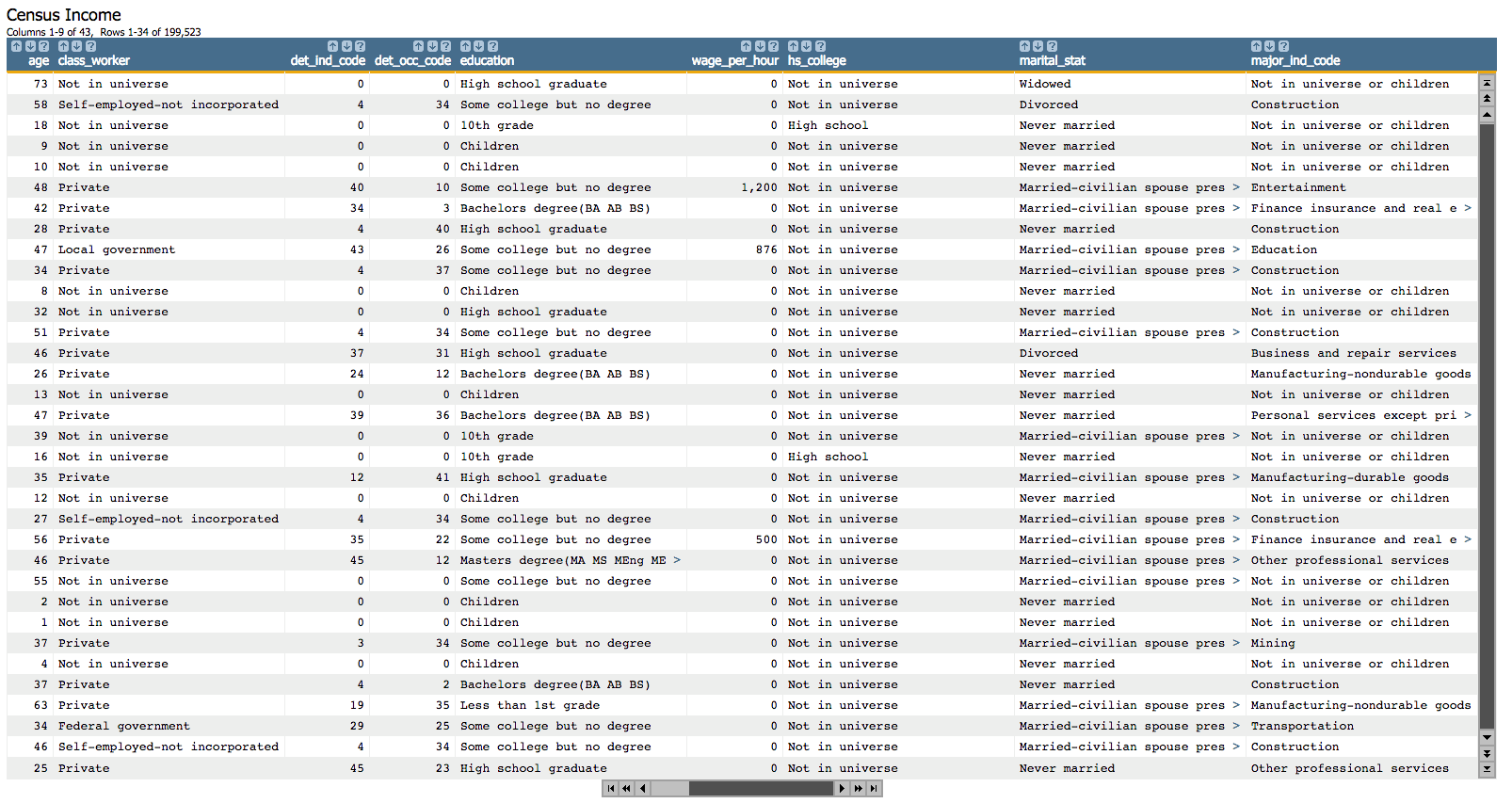2. Select only those rows where `wage_per_hour` is not equal to 0, since we only want to do the regression for those people who have a job.
``<sel value="(wage_per_hour<>0)"/>``
3. Since we're using `age` as a predictor, let's see if it has a linear relationship to `wage_per_hour`, our response.
1. For visualization purposes, we can look at the relationship between the average wage per hour and age.

Let's first calculate the average wage per hour grouping by age. We'll use the function `g_avg(G;S;X)` and we'll specify the `age` column for the `G` parameter (we're grouping by age) and `wage_per_hour` as the `X` parameter (since we want to calculate the average wage per hour). We'll omit the `S` parameter since we want to consider all rows in the table.

``````<willbe name="avg_wage_per_hour_age" value="g_avg(age;;wage_per_hour)"
format="dec:2"/>``````
2. Click Chart > Line.
3. Drag the `age` column to the DATA (X-AXIS) area.
4. Drag the `avg_wage_per_hour_age` column to the DATA (Y-AXIS) area.
5. Click Update.Since we can see from this chart that the relationship between `age` and `wage_per_hour` has a quadratic form, we will include the square of the age as one of the predictors.

4. Let's create a column containing the square of the value in the `age` column, which we will use as one of the predictors.
``<willbe name="age_sq" value="age^2"/>``
5. Since we're also using `edu_year` as a predictor, let's see if it has a linear relationship to `wage_per_hour`.
1. For visualization purposes, we can look at the relationship between the average wage per hour and years of education.

Let's first calculate the average wage per hour grouping by years of education. We'll use the function `g_avg(G;S;X)` and we'll specify the `edu_year` column for the `G` parameter (we're grouping by years of education) and `wage_per_hour` as the `X` parameter (since we want to calculate the average wage per hour). We'll omit the `S` parameter since we want to consider all rows in the table.

``<willbe name="avg_wage_per_hour_edu_year" value="g_avg(edu_year;;wage_per_hour)" format="dec:2"/>``
2. Click Chart > Line.
3. Drag the `edu_year` column to the DATA (X-AXIS) area.
4. Drag the `avg_wage_per_hour_edu_year` column to the DATA (Y-AXIS) area.
5. Click Update.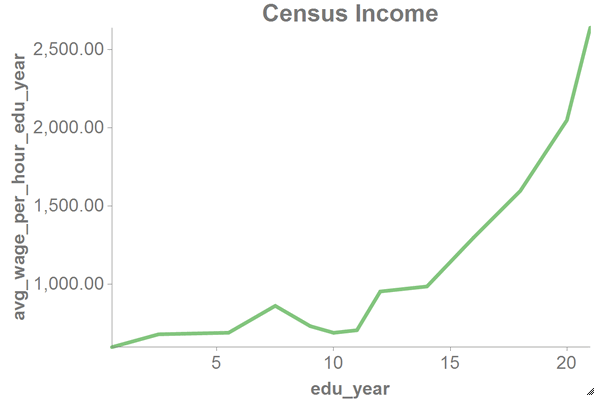We can see from this chart that the relationship between `edu_year` and `wage_per_hour` has a linear form.

6. Now, let's show only those columns that we are going to use in the model (as well as those we used in the charts from the previous steps).
``````<colord cols="avg_wage_per_hour_age,avg_wage_per_hour_edu_year,
wage_per_hour,age,edu_year,instance_weight,age_sq"/>``````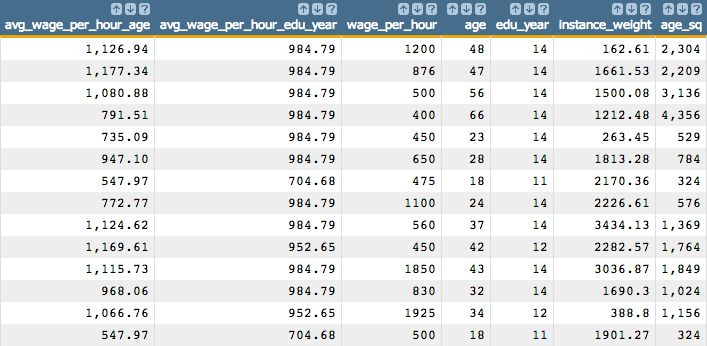7. Next, we fit our model on the three predictors as well as the response that we have selected using the `g_wlsq(G;S;Y;W;XX)` function.
``<willbe name="model_wlsq" value="g_wlsq(;;wage_per_hour;instance_weight;1 age age_sq edu_year)"/>``
Note: As the first element of `XX`, we specify the special value 1 for the constant (intercept) term in the linear model.

This creates a column named `model_lsq` that contains the results of the least squares regression: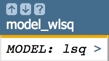Clicking on the `>` opens a window containing a summary of the model output: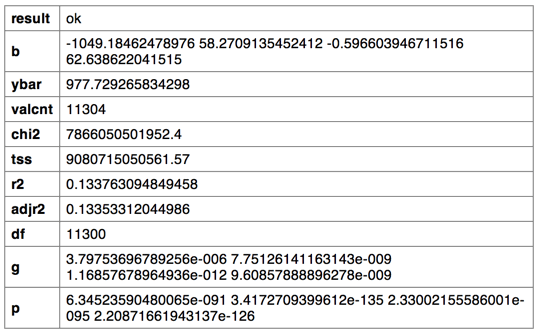8. We can then obtain the predicted value of the linear model using the `score(XX;M;Z)` function.
``````<note>OBTAIN MODEL SCORE WITH SCORE FUNCTION</note>
<willbe name="pred" value="score(1 age age_sq edu_year;model_wlsq;)" format="dec:7"/>``````
Note: We specify `format="dec:7"` so that our results show with 7 decimal places.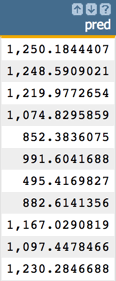9. To obtain the coefficients of the linear model, we can use the `param(M;P;I)` function.

In the following Macro Language code, we obtain the parameters for the intercept (`b0`) and the first two variables (`b1` and `b2`).

``````<note>OBTAIN MODEL COEFFICIENTS</note>
<willbe name="b0" value="param(model_wlsq;'b';1)" format="dec:7"/>
<willbe name="b1"value="param(model_wlsq;'b';2)" format="dec:7"/>
<willbe name="b2" value="param(model_wlsq;'b';3)" format="dec:7"/>``````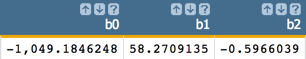10. We can also obtain the p-values of the coefficients using the `param(M;P;I)` function. We will use these to conduct the variable selection later in the analysis.

In the following Macro Language code, we obtain the p-value for the intercept (`p0`) and the first two variables (`p1` and `p2`).

``````<note>OBTAIN P-VALUES</note>
<willbe name="p0" value="param(model_wlsq;'p';1)"/>
<willbe name="p1" value="param(model_wlsq;'p';2)"/>
<willbe name="p2" value="param(model_wlsq;'p';3)"/>``````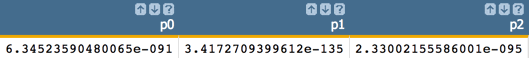11. However, one might want to obtain all of the coefficients in one column and the p-values in another, which can be achieved with the following Macro Language code:
``````<note>CALCULATE ALL COEFFICIENTS IN ONE COLUMN AND P-VALUES IN ANOTHER</note>
<willbe name="var_names_1" value="'intercept,age,age_sq,edu_year'"/>
<willbe name="temp_i_1" value="mod(i_(1);4)"/>
<willbe name="i_1" value="if(temp_i_1=0;4;temp_i_1)"/>
<willbe name="b_1" value="param(model_wlsq;'b';i_1)" format="dec:7"/>
<willbe name="p_1" value="param(model_wlsq;'p';i_1)"/>
<willbe name="var_name_1" value="csl_pick(var_names_1;i_1)"/>``````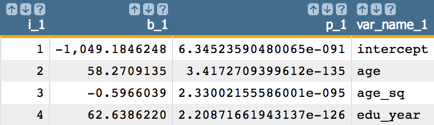Note: The number of coefficients and p-values we obtain from the linear model corresponds to the number of variables in our analysis. So, for our example, we obtain 4 coefficients and 4 p-values, which correspond to the intercept plus the 3 predictors.
12. Using the `param(M;P;I)` function, we can obtain various statistics for this model such as the degrees of freedom of the model, residual sum of squares, mean squared error, number of observations, average of `Y`, R2, and adjusted R2.
``````<note>OBTAIN VARIOUS MODEL STATISTICS</note>
<willbe name="dof" value="param(model_wlsq;'df';)"/>
<willbe name="sse_temp" value="(wage_per_hour-pred)^2"/>
<willbe name="sum_sq_resids" value="g_sum(;;sse_temp)" format="dec:7"/>
<willbe name="mean_sq_err" value="sum_sq_resids/dof" format="dec:7"/>
<willbe name="num_observations" value="param(model_wlsq;'valcnt';)"/>
<willbe name="avg_y" value="param(model_wlsq;'ybar';)" format="dec:7"/>
<willbe name="R_squared" value="param(model_wlsq;'r2';)" format="dec:7"/>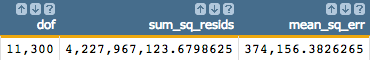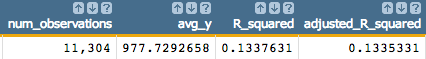13. We can calculate the AIC using the following Macro Language code:
``````<note>CALCULATE AIC</note>
<willbe name="sum_log_likelihood" value="loge(sum_sq_resids/num_observations)"/>
<willbe name="num_of_var" value="3"/>
<willbe name="AIC" value="2*num_of_var+num_observations*sum_log_likelihood"/>``````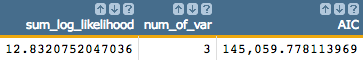14. Finally, we can visualize the results of the weighted least squares regression using the 1010data Chart Builder.
1. Click Chart > Scatter.
2. Drag the `age` column to the DATA (X-AXIS) area.
3. Drag the `wage_per_hour` and `pred` columns to the DATA (Y-AXIS) area.
4. Click Update.

The results should look similar to the following chart: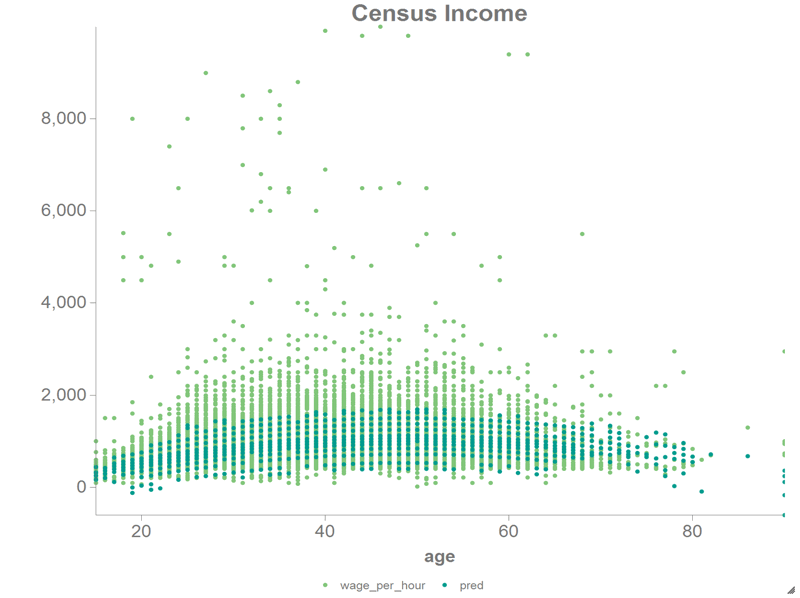If you want to see how to calculate the standard error of the coefficients or how to chart the residual plot, QQ plot, PP plot, or how to plot the predicted value against the original response, see the Least Squares Regression tutorial.